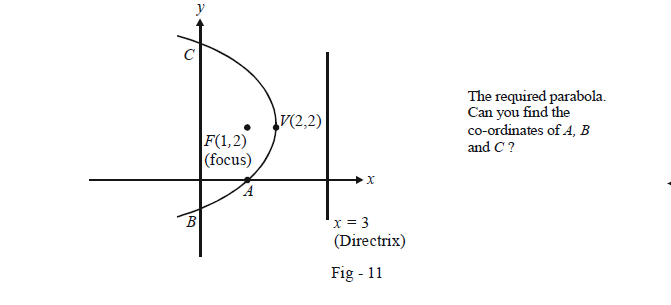# Basic Equations of Parabolas

Go back to  'Parabola'

As just discussed, a parabola is the locus of a moving point P such that its distance from a fixed point (the focus F) is always equal to its distance from a fixed line L = 0, the directrix.

For a start (and to make things easier), we assume F to be the point (a, 0) and the line L to be $$L \equiv x + a = 0.$$ The origin then lies mid-way between F and L. There’s no loss of generality in doing so since howsoever F and L may lie in the plane, we can always (by a suitable choice of the axes) make the origin of our axes lie half-way between F and L and the y-axis parallel to L.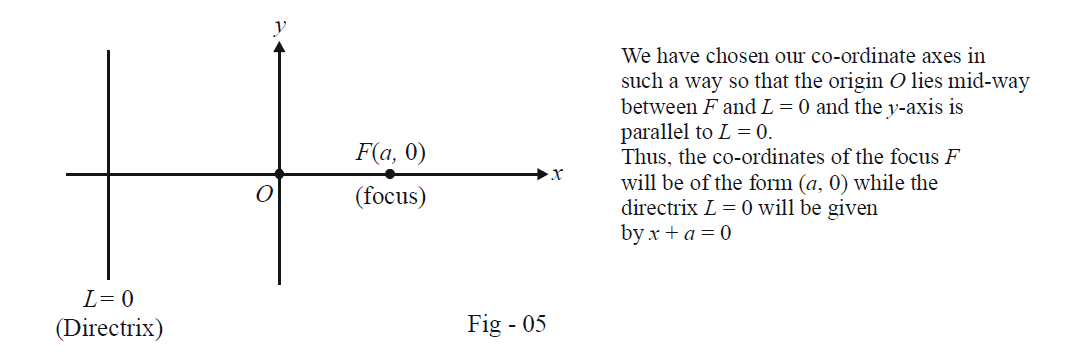Observe that since the origin O is equidistant from F and L = 0, it too lies on the parabola. Thus, our parabola will pass through the origin.

To find the equation representing the parabola, we assume the co-ordinates of point P lying on it as (x, y). Thus,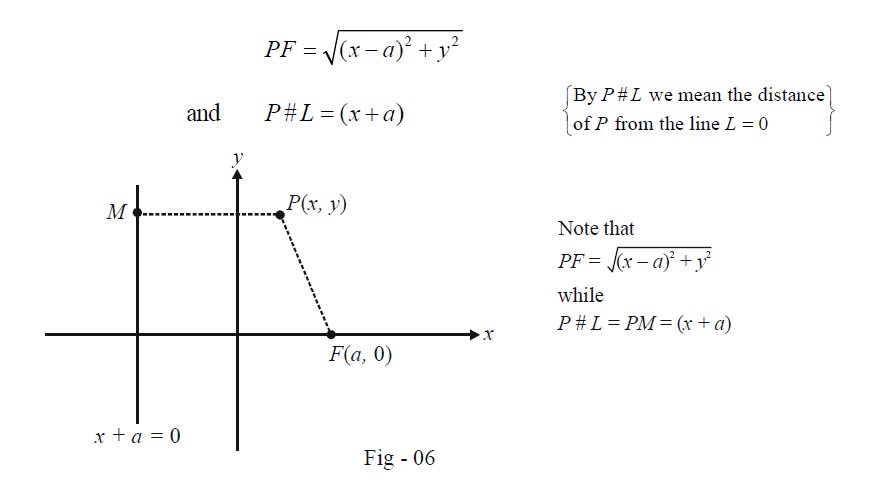By the definition of a parabola, these two distances must always be equal so that

\begin{align}& \qquad \;\; {(x - a)^2} + {y^2} = {(x + a)^2}\\&\Rightarrow\quad \fbox{$${y^2} = 4ax$$} \,\,\,:\,\,\,{\rm{Equation of a parabola}}\end{align}

This is referred to as the standard equation of the parabola with focus F(a, 0) and directrix $$L \equiv x + a = 0$$ .

When we actually plot the curve $${y^2} = 4ax,$$ we obtain the following shape. Any point on this curve is always equidistant from F (a, 0) and $$L \equiv x + a = 0$$ .The line y = 0 which passes through the focus F(a, 0) as is perpendicular to the directrix x + a = 0 will be termed the axis of the parabola. The origin, which is halfway between the focus and the directrix, will be termed the vertex of the parabola.

It should now be obvious for you to deduce what the curve $${y^2} = 4ax,$$ will look like if a < 0. In this case, the focus F(a, 0) lies on the negative x-axis while the directrix x + a = 0 is to the right of the origin: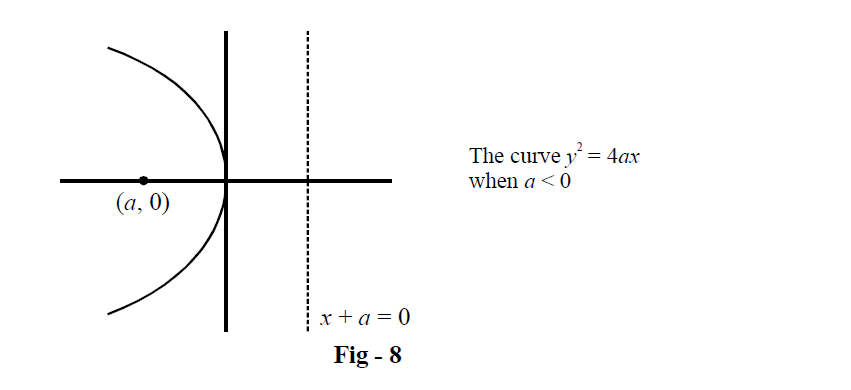We can also deduce what the curve $${x^2} = 4ay$$ will look like; just interchange the role of x and y. The focus and directrix will change accordingly as described in the figure below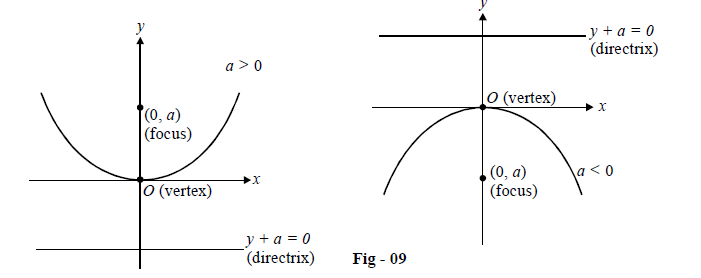In fact, we can now generalise this discussion to a parabola with vertex at V (h, k) and the axis parallel to the x-axis or the y-axis. Note that in these parabolas, the focus lies at a distance of |a| from the vertex along the axis of the parabola :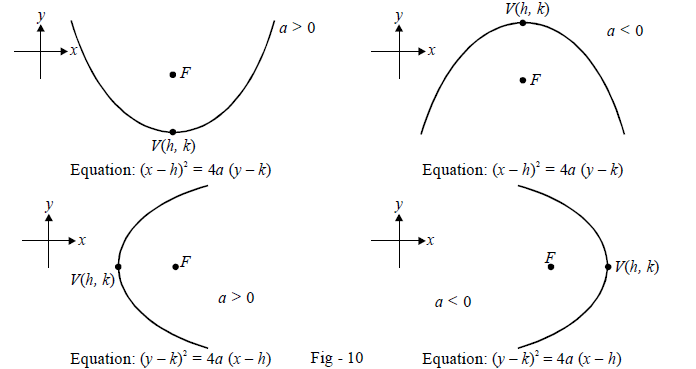Example - 1

Plot the parabola given by the equation $${y^2} - 4y + 4x - 4 = 0$$ .

Solution: The given equation can be rearranged as

${(y - 2)^2} = - 4(x - 2)$

This represents a parabola with vertex V(2, 2) and opening towards the left (the fourth case above in Fig - 10) because a = –1 (negative).

The focus will lie at a distance 1 unit to the left of (2, 2), i.e. ,at (1, 2). The directrix will lie 1 unit to the right of (2, 2), i.e. it will be x = 3.

The following figure shows this parabola: